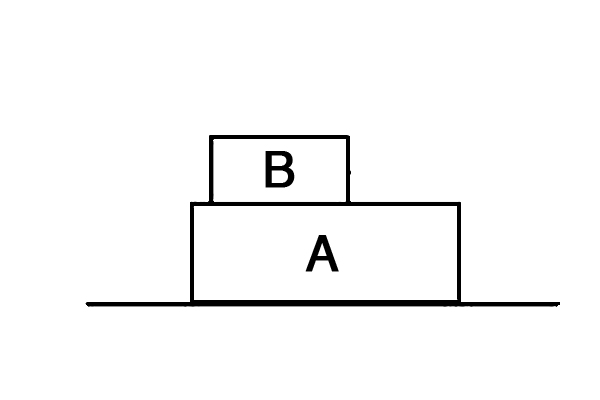Classical Mechanics

# Newton's Third Law

Two boats $$A$$ and $$B,$$ connected with a string, are floating on a calm lake. The mass of $$A$$ and $$B$$ are $$100 \text{ kg}$$ and $$150 \text{ kg},$$ respectively, and the distance between them is $$30 \text{ m}.$$ If the string is pulled from boat $$A$$ with a constant force and the two boats meet in $$20 \text{ seconds},$$ what is the distance that boat A moves?

Suppose that there is no friction whatsoever.

A $$72 \text{ kg}$$ package lying on the ground is connected to a massless rope that runs over a frictionless tree limb. If a $$40 \text{ kg}$$ monkey climbs up the other side of the rope, what is the magnitude of the minimum acceleration at which the monkey should climb for the package to be lifted off the ground?

The gravitational acceleration is $$g= 10 \text{ m/s}^2 .$$As shown in the diagram above, two objects $$A$$ and $$B$$ that have respective masses of $$m_A$$ and $$m_B$$ are on a horizontal plane. The force with which the plane supports $$A$$ is $$2$$ times the force with which $$A$$ supports $$B.$$

Find $$m_A/m_B$$.

For Newton’s third law of motion to hold, what must be the state of motion of the two objects involved?

You are holding onto a $$100~\mbox{kg}$$ basket of fish hanging over the side of a boat. What is the minimum force in Newtons you need to exert to keep the basket from falling back down into the ocean?

Details and assumptions

• The acceleration of gravity is $$-9.8~\mbox{m/s}^2$$.
×

Problem Loading...

Note Loading...

Set Loading...